Subsections

## 3.2.1 Multiple moving interfaces

In order to model multiple moving interfaces in the LS method from Section 1.4.1, two separate LS systems are analyzed. One system is used for the Si-SiO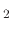interface, which is an etch-like process, proceeding with a relative negative velocity, while a separate system is used for the SiO-ambient interface, which is a deposition-like process, proceeding with a relative positive velocity. Three different scenarios can lead to different methods by which the LS system should be described: An initial LS description of one interface, which will grow to two interfaces at the onset of oxidation, pre-grown material such as a native oxide layer which will grow further during the oxidation process, and the existence of a mask layer, which should affect the movement of both interfaces.

### 3.2.1.1 One initial LS description

When the initial geometry is described by a single material interface, which must split and simultaneously move in opposite directions, the LS systems are straightforwardly implemented. If we label the initial interface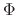, then the split is performed by simply introducing a new LS interface. We now have a system with LS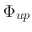which should move in the positive deposition-like direction andwhich should move in the negative etching-like direction. Figure 3.1 depicts such a scenario for a 500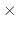500 geometry where the top surface moves at a rate of 0.25grid/time and the bottom surface moves at a rate of -0.75grid/time for 10 time units. Therefore,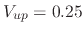and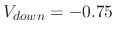is set and the LS equations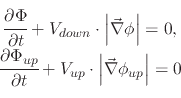(88)

are solved. When processing is complete,is added to the top of the stack in theLS system.

Figure 3.1: Modeling approach when a single LS interface is split into two interfaces, which move in opposite directions for a 500500 geometry moving at velocities 0.25 and -0.75 for the top and bottom surfaces, respectively.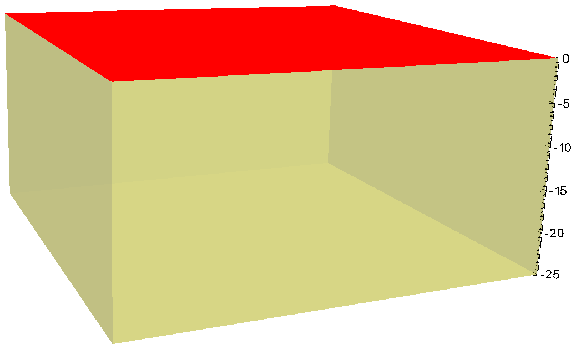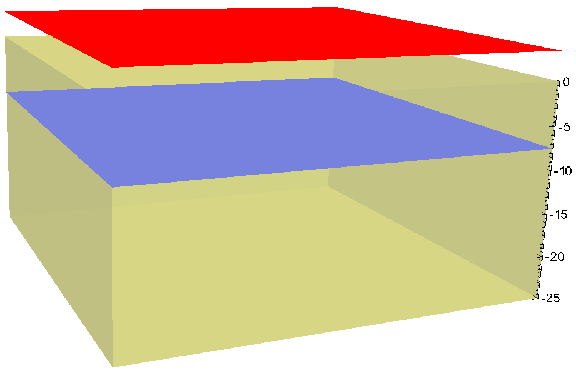(a) Initial interface. (b) Initial interface. (c) Initial interface.

### 3.2.1.2 LS describes pre-existing native material

When the initial geometry involves two level set interface systems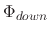andand the goal of the simulation is to move them downward and upward, respectively, no interface splitting is required. Only the separation of the two interfaces into two different LS systems is required. Therefore, assuming the initial interface system, then we introduce a second LS system. The top LS interface fromis removed, while the bottom interface fromis also removed. The two systems are now separated as shown in Figure 3.2a and Figure 3.2b, respectively. Figure 3.2c depicts the scenario after the following LS equations were processed for the individual surfaces on a 500500 geometry: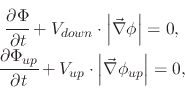(89)

where the top surface moves at a rate of 0.25grid/time and the bottom surface moves at a rate of -0.75grid/time for 10 time units:and. When processing is complete,is added to the top of the stack in theLS system resulting once again in the LS systemwhich contains two LS interfaces.

Figure 3.2: Modeling approach when the initial LS geometry contains two interfaces which need to be moved in opposite directions, for a 500500 geometry at velocities 0.25 and -0.75 for the top and bottom surfaces, respectively.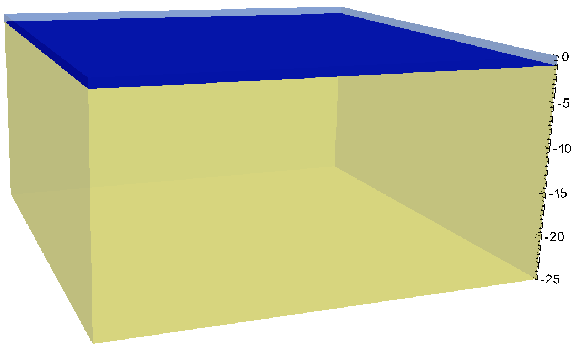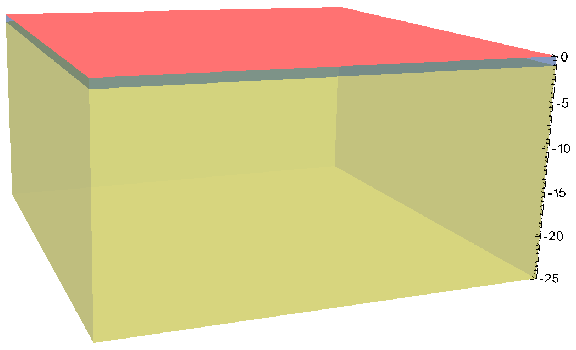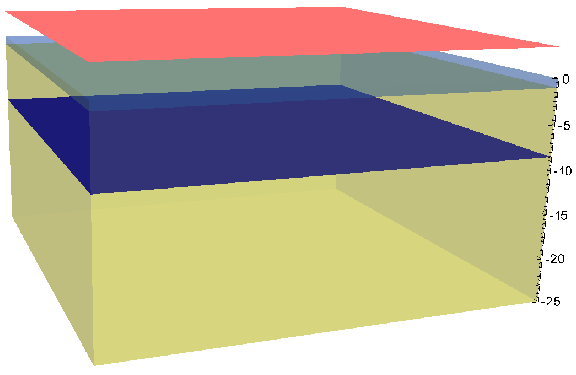(a) Initial interface. (b) Second interface. (c)after processing.

### 3.2.1.3 LS describes existence of a mask layer

When a mask layer is introduced into a system where a material is grown in such a way that two LS interfaces need to be simultaneously moved in opposite directions, the mask layer influences the movement of both level sets. Therefore, when the LS system is split, such as those from Figure 3.1 and Figure 3.2, the mask layer must remain in both LS systems. Another aspect which must be considered is the manner in which the different LS interfaces are labeled. This will be addressed in Section 3.2.2, while here only the initial separation of materials to form the two LS systems will be described. Assuming we start with a LS description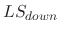which includes two surfaces. One describes the location of the mask and the second the location of the material which will be grown and is located below the mask. A second LS description can now be initiated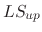, which only holds the mask LS from.contains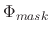andand it is a simple matter of moving the desired interface by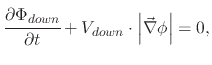(90)

which is analogous to an etching process with a mask, described in . An additional LS interface,is added to thestack at the interface between the mask and the bottom-most material. Therefore, when a positive velocity is applied toaccording to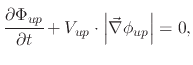(91)

the mask will act as a block, similar to the way it blocks an etching process. Figure 3.3 shows this event for a geometry 15155 where the lower surface is prepared to be etched'' while the top surface is prepared to undergo mask-blocking deposition''.
Figure 3.3: Modeling approach when the initial LS geometry contains two interfaces, one a mask and one a surface to be grown. A 15155 geometry is used.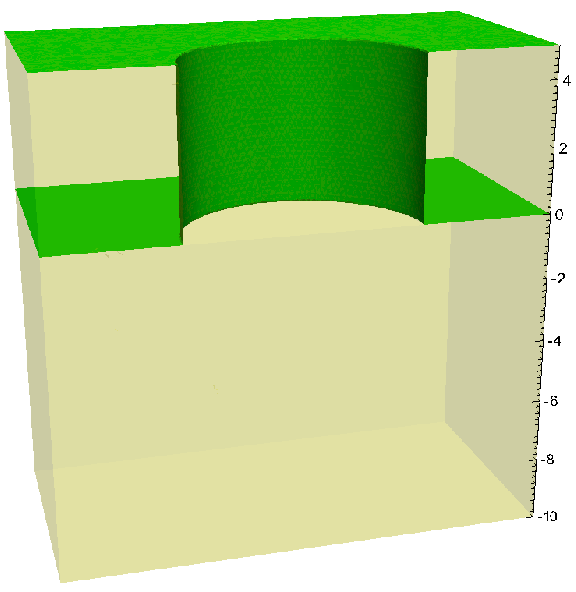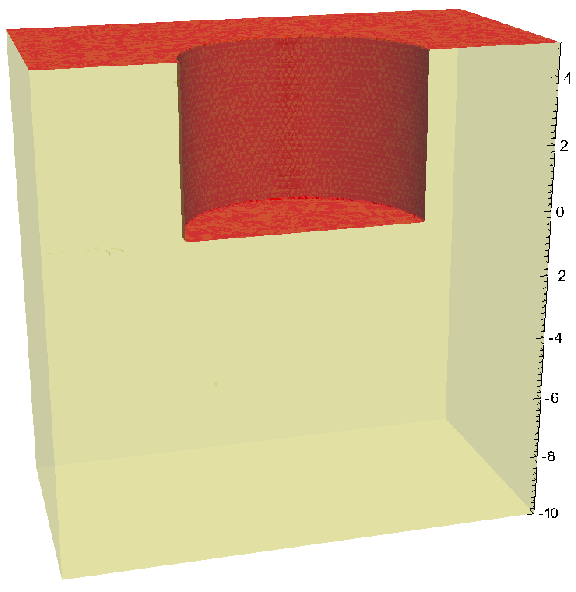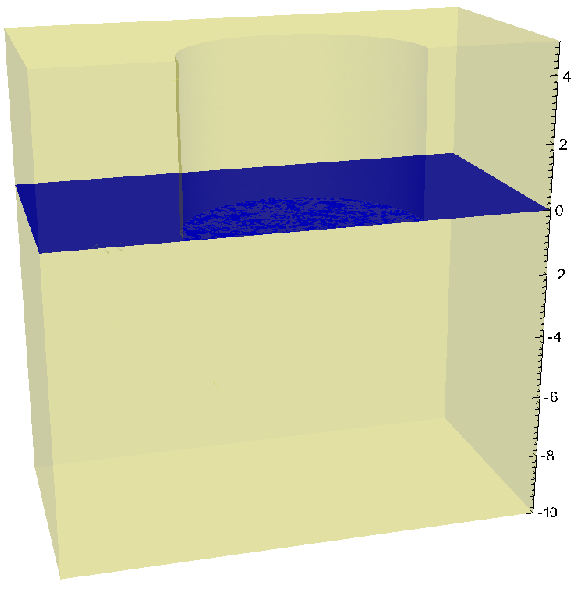(a) Initial mask surface. (b) Initial. (c) Initial.

L. Filipovic: Topography Simulation of Novel Processing Techniques